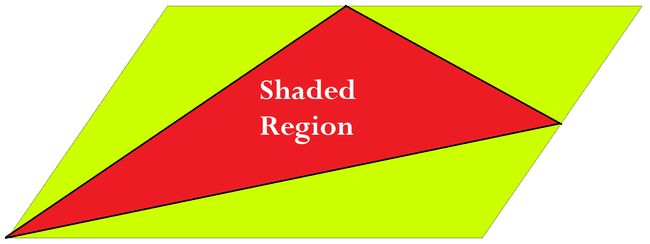Geometry Level 2In the given parallelogram, the midpoints of two adjacent sides of the parallelogram are joined and then connected to the opposite vertex to form a triangle.

What fraction of the total parallelogram is the shaded area?